Vectors and conics

This free course is available to start right now. Review the full course description and key learning outcomes and create an account and enrol if you want a free statement of participation.

Free course

1.3 Parallel and perpendicular lines

We often wish to know whether two lines are parallel (that is, they never meet) or perpendicular (that is, they meet at right angles).

Two distinct lines, y = m1x + c1 and y = m2x + c2, are parallel if and only if they have the same gradient; that is, if and only if m1 = m2. For example, the lines y = −2x + 7 and y = −2x − 3 are parallel since both have gradient −2, whereas the lines y = −2x + 7 and y = 2x − 3 are not parallel since their gradients are not equal (they are −2 and 2, respectively).

Two lines ℓ1 and ℓ2 with equations y = m1x + c1 and y = m2x + c2, respectively, where m1 and m2 are both non-zero, are perpendicular if and only if m1m2 = −1. If the lines are perpendicular, then one (ℓ1, say) must slope up from left to right and the other (ℓ2, say) must slope down from left to right, as shown below.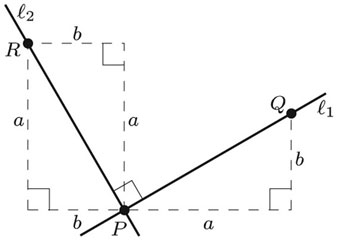Let the lines intersect at P, and let Q be a point on ℓ1 to the right of P. Suppose that Q is a units to the right of P and b units up from P, as illustrated above. Let R be the point on ℓ2 obtained by rotating PQ anticlockwise through an angle of/2; then R is b units to the left of P and a units up from P, as shown.

Then the gradient of ℓ1 is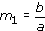and the gradient of ℓ2 is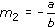It follows that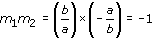The proof of the converse is similar.

Two distinct lines with equations y = m1x + c1 and y = m2x + c2, where m1 and m2 are both non-zero, are

• parallel if and only if m1 = m2 and c1c2;

• perpendicular if and only if m1m2 = −1.

Example 1

Determine which of the following lines are parallel, and which are perpendicular to each other.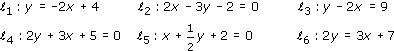The gradients of the given lines are, respectively:

−2,, 2,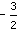, −2 andThus the lines ℓ1 and ℓ5 are parallel, and the lines ℓ2 and ℓ4 are perpendicular.

Example 3

Determine which of the following lines are parallel, and which are perpendicular to each other.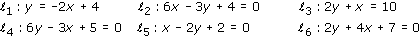Since the gradient of a line whose equation is in the form y = mx + c is m, the gradients of the given lines are, respectively:

−2, 2,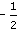,,and −2

Thus ℓ1 and ℓ6 are parallel, and ℓ4 and ℓ5 are parallel; ℓ1 and ℓ4 are perpendicular, ℓ1 and ℓ5 are perpendicular, ℓ2 and ℓ3 are perpendicular, ℓ4 and ℓ6 are perpendicular, and ℓ5 and ℓ6 are perpendicular.

M208_1